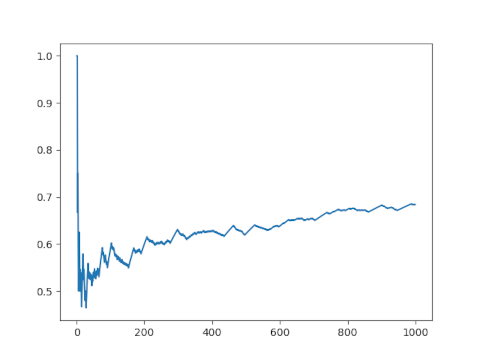# Pascal’s triangle mod row number

Almost all binomial coefficients are divisible by their row number.

This is a theorem from . What does it mean?

If you iterate through Pascal’s triangle, left-to-right and top-to-bottom, noting which entries C(m, k) are divisible by m, the proportion approaches 1 in the limit.

The author proves that the ratio converges to 1, but doesn’t say anything about the rate at which it approaches 1. By writing a little Python script we can observe how the ratio approaches 1.

First, let’s create a generator that will let us walk through Pascal’s triangle.

```    def pascal():
m, k = 0, 0
while True:
yield (m, k)
k += 1
if k > m:
m += 1
k = 0
```

We can use this generator to illustrate Pascal’s triangle mod row number.

```    from math import comb

p = pascal()
m, k = next(p)
while m < 10:
ch = "*"
if m > 0 and comb(m, k) % m == 0:
ch = "0"
print(ch, end="")
if m == k:
print()
m, k = next(p)
```

This produces the following.

```    *
00
*0*
*00*
*0*0*
*0000*
*0***0*
*000000*
*0*0*0*0*
*00*00*00*
```

The theorem says that as we keep going, the proportion of 0’s in a diagram like the one above approaches 1.

Now let’s plot the proportion of zeros as we traverse Pascal’s triangle mod row number.

```    N = 1000
x = np.zeros(N)
p = pascal()
for n in range(N):
m, k = next(p)
if m > 0 and comb(m, k) % m == 0:
x[n] = 1
y = np.arange(N)
plt.plot(y, x.cumsum()/y)
plt.show()
```

Here’s what we get.The ratio doesn’t seem to be converging to 1. If I had to guess, I might say it’s converging to 0.7 by looking at this plot. But if we go further out and switch to a log scale it seems more plausible that the sequence is converging to 1, albeit very slowly.Heiko Harborth. Divisibility of Binomial Coefficients by Their Row Number. The American Mathematical Monthly, Jan. 1977, Vol. 84, No. 1, pp. 35–37.

## 2 thoughts on “Pascal’s triangle mod row number”

1. Jonathan

That is one of the slowest convergences I’ve ever seen!

It makes me wonder if its approximate behavior has a bunch of log(log(log(n)))-type terms in it, which are what scare me away from Erdos’ and Ramanujan’s work.

2. Michael Albert

Amusingly, you can build the C(m,k) computation into the generator:

def pascal():
m, k, c = 0, 0, 1
while True:
yield (m, k, c)
c *= m-k
k += 1
if (k > m):
m += 1
k = 0
c = 1
else:
c //= k